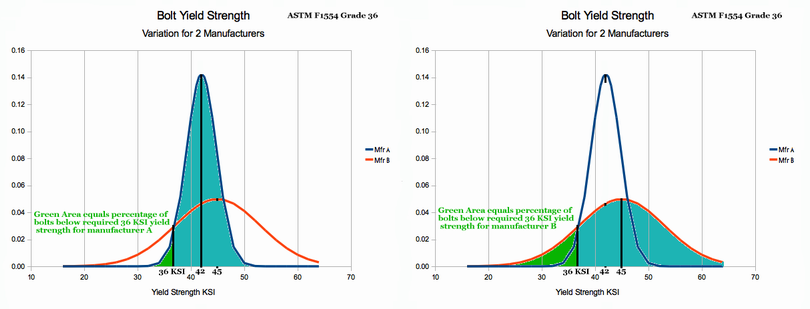# What is Statistics and Probability About?

### For the Salvation of Many, by Saint Paul

Music 1: Piano Etude (public domain)

Section Goals:

1. Acquaint Student with Concept and Definition of Probability.
2. Acquaint Student with Concept and Definition of Statistics. Draw distinction between Probability & Statistics.

Introduction:
Statistics is a set of mathematical tools for analysing and presenting numeric data. The numeric data might concern a new drug, the failures of aircraft electronics or the accuracy of the army's newest cannon. Often, large amounts of such data overwhelm the mind and leave it unable to draw meaningful conclusions. In such circumstance, statistics provides a way of thinking and a set of tools that can help.

Learning Statistics:
Statistics is usually presented as dry, mathematical formulas that are explained in arcane words spoken by professors. In this series of lessons, St. Paul will explain much, using ordinary English. But you must do your part!

Your part is to follow the examples in detail. Work them yourself and compare your answer with Saint Paul's. Where homework is requested, do the work and compare answers with the ones that Paul provides. If you get "stumped", send an e-mail to paul-watson@sbcglobal.net . If Paul is not absolutely swamped, he will reply to your question.

Some lessons are marked OPTIONAL. These may be safely skipped if the student likes. All others should be followed carefully. if YOU get tired or saturated, you'd better quit for the night. Down stream progress requires a full understanding of the material. Learning statistics is a journey, not a "quickie".

Note: Unfortunately, Statistics requires quite a lot of "number grinding". You will need a calculator. A spreadsheet can help a lot. You have to grind through the numbers for the knowledge to "sink into your head". But try to see the big patterns. Don't just grind through creating the table in Chapter 1. When that table is done, look at the pattern of how each row is computed. They are all the same, except for the number of ways the desired result can be achieved. Grind through the detail, look for the patterns. I find statistics quite beautiful and useful. I hope it works for you as well. If not, well there is always mechanics of solids, or art!

Definitions & Concepts:
What is Probability?
Probability is the likelihood of a specific event occurring. It is usually expressed as a percentage. If a gambler is throwing a single die and wants to throw a 5, then what is the probability of success? Answer: An honest die has 6 sides, equally likely. So there is one side out of 6 that meets the gambler's need. The probability of success is thus 1/6. On a calculator, 1 divided by 6 = .166667 or 16.7%. This means that out of 100 throws of a die, a gambler would get about 16 successes.

No one can predict the outcome of a single throw of the dice; however, statistics can predict the percentage of various outcomes that result from a million throws of the dice. For 100 throws, statistics can provide a good approximation. The tendency of the result for a very large number of trials is what probability describes.

What is Statistics?
Wikipedia says statistics is "The collection of numerical data, the analysis of numerical data and the presentation of numerical data." Judgements on the quality of Picasso's paintings are thus outside the realm of statistics, but interpretation of numerical data concerning how many people like his art CAN be treated with statistics. Statistics deals with numbers -- not value judgements.

Very often, statistics deals with probabilities stacked on top of probabilities. For example, consider 2 dice thrown by a gambler. Assume we want to know the probability of various outcomes. Dealing with the interaction of two dice is an example of probabilities stacked on top of probabilities and such analysis is statistics. Chapter 1 analyses the two dice problem, demonstrates probabilities stacked on probabilities and introduces the Probability Density Function (PDF for short).

Course Outline:
The following links provide a course in statistics. To be effective, you should start with chapter 1. Take the lessons in order and study each thoroughly. Once you have mastered the material, you can use the chapter titles to pick review topics of interest to you. Good luck to you and I hope you enjoy statistics and find many uses for it.

Contact the author
paul-watson@sbcglobal.net by e-mail.